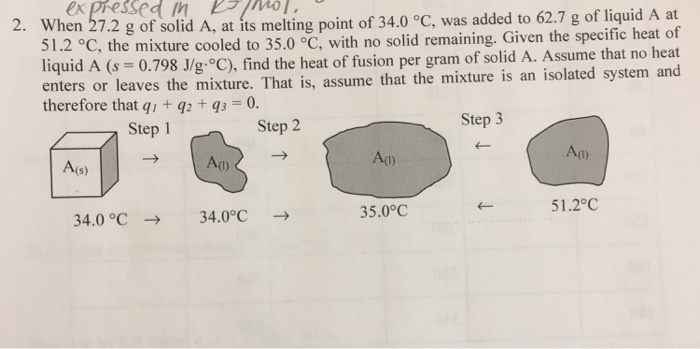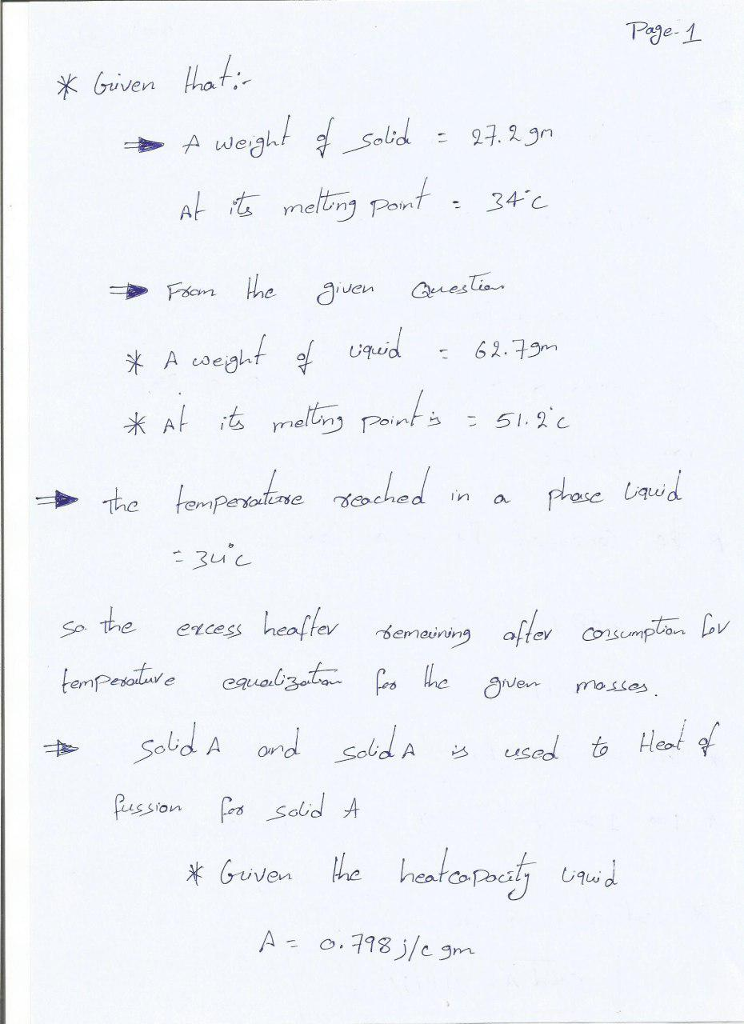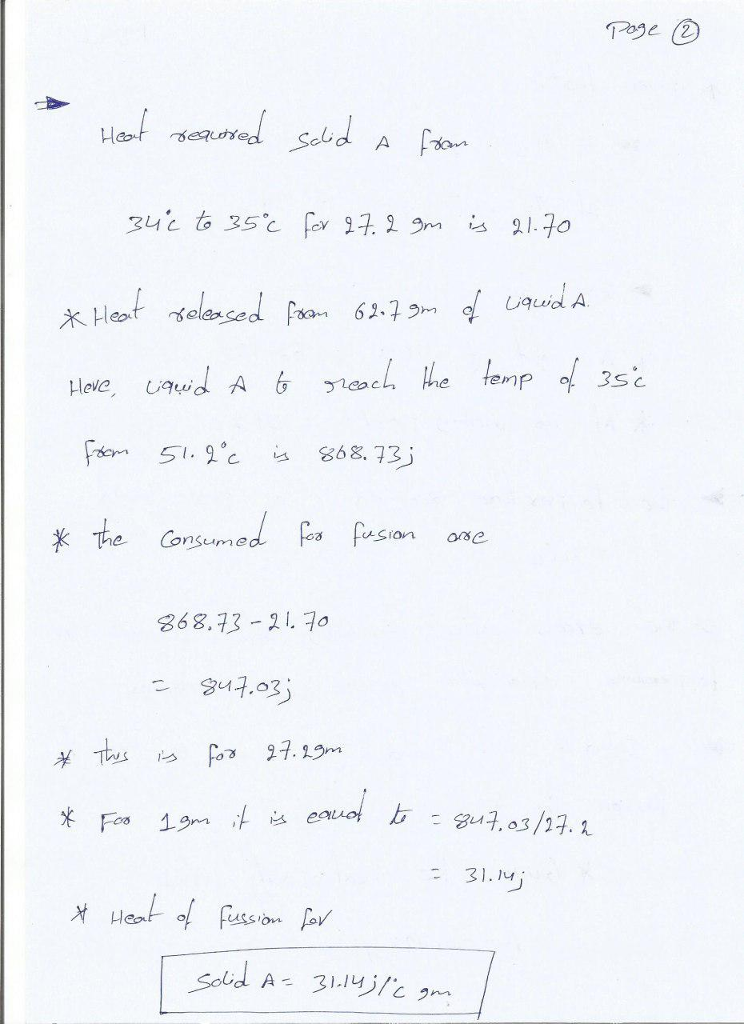# expressed m K /01, 27.2 g of solid A, at its melting point of 34.0 °C,...expressed m K /01, 27.2 g of solid A, at its melting point of 34.0 °C, was added to 62.7 g of liquid A at the mixture cooled to 35.0 °C, with no solid remaining. Given the specific heat of id A (s = 0.798 J/g·°C), find the heat of fusion per gram of solid A. Assume that no heat enters or leaves the mixture. That is, assume that the mixture is an isolated system and 2. When therefore that qi + g2t g30. Step1 Step 2 Step 3 A(s) Au Ao 34.0 oC34.0°C 35.0°C 51 .2°C#### Earn Coin

Coins can be redeemed for fabulous gifts.

Similar Homework Help Questions
• ### The specific heat capacity of solid lead is 0.159J/g°C, the molar enthalpy of fusion is 5.0kJ/mol, and the melting point of lead is 328°C

The specific heat capacity of solid lead is 0.159J/g°C, the molar enthalpy of fusion is 5.0kJ/mol, and the melting point of lead is 328°C. Calculate the total energy required to change 100g of lead at 25°C to molten lead at it's melting point. I will be happy to critique your work or thinking. See the other posts in this series for hints. Fair enough, the least I can do is try it. Basically I will find the heat required to...